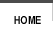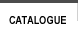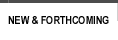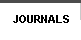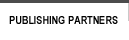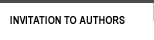sitemap | contact usISBN Author Title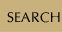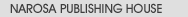Book SeriesJournalsBook Proposal FormWriting a BookUsing Published MaterialRights and PermissionsExamination Copies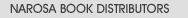List of PublishersBargains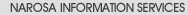Services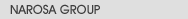About NarosaHistoryMissionGroup CompaniesOur StrengthAlliances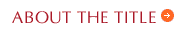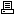view in print mode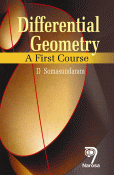Differential Geometry: A First Course Author(s): D. Somasundaram ISBN:    978-81-7319-546-4  E-ISBN:    Publication Year:   Reprint 2019 Pages:   470 Binding:   Paper Back Dimension:   160mm x 240mm Weight:   700 Textbook

 About the book Differential Geometry: A First Course is an introduction to the classical theory of space curves and surfaces offered at the Graduate and Post- Graduate courses in Mathematics. Based on Serret-Frenet formulae, the theory of space curves is developed and concluded with a detailed discussion on fundamental existence theorem. The theory of surfaces includes the first fundamental form with local intrinsic properties, geodesics on surfaces, the second fundamental form with local non-intrinsic properties and the fundamental equations of the surface theory with several applications. Key Features Motivation of different concepts  Outline of the methods of proofs before giving details  Simple but interesting immediate illustrations in each section after the theory  Illuminating miscellaneous examples  Illustrations of the methods of proofs of the fundamental theorems of space curves and surfaces  Exercises with hints Table of Contents Theory of Space Curves: Introduction / Representation of space curves / Unique parametric representation of a space curve / Arc  length / Tangent and Osculating Plane / Principal normal and binormal / Curvature and Torsion / Behaviour of a curve near one of its points / Curvature and torsion of the curve of intersection of two surfaces / Contact between curves and surfaces / Osculating circle and osculating sphere / Locus of centres of spherical curvature / Tangent surfaces, Involutes and evolutes / Betrand curves / Spherical Indicatrix / Intrinsic equations of space curves / Fundamental Existence Theorem for space curves / Helices / Examples 1 / Exercises 1 / The First Fundamental Form and Local Intrinsic Properties of a Surface: Introduction / Definition of a surface / Nature of points on a surface / Representation of a surface / Curves on surfaces / Tangent plane and surface normal / The general surface of revolution / Helicoids / Metric on a surface / Direction coefficients on a surface / Families of curves / Orthogonal Trajectories / Double Family of curves / Isometric correspondence / Intrinsic properties / Examples II / Exercises II / Geodesics on a Surface: Introduction / Geodesics and their differential equations / Canonical geodesic equations / Geodesics on surfaces of revolution / Normal property of geodesics / Differential equations of geodesics using normal property / Existence theorems / Geodesic parallels / Geodesic curvature / Gauss  Bonnet theorem / Gaussian Curvature / Surfaces of constant curvature / Conformal mapping / Geodesic mapping / Examples III / Exercises III / The Second Fundamental form and local Non - Intrinsic Properties of Surfaces: Introduction / The second fundamental form / The Classification of points on a surface / Principal curvatures / Lines of curvature / The Dupin indicatrix / Developable surfaces / Developables associated with space curves / Developables associated with curves on surfaces / Minimal surfaces / Ruled surfaces / Three fundamental forms / Examples IV / Exercises IV / The Fundamental Equations of Surface Theory: Introduction / Tensor notations / Gauss equations / Weingarten Equations / Mainardi  Codazzi equations / Parallel Surfaces / Fundamental existence theorem for surfaces / Examples V / Exercises V Audience Graduate and Postgraduate Students

# var message="For Bargains from Narosa Book Distributors, " var neonbasecolor="black" var neontextcolor="red" var neontextcolor2="red" var flashspeed=100 // speed of flashing in milliseconds var flashingletters=2 // number of letters flashing in neontextcolor var flashingletters2=2 // number of letters flashing in neontextcolor2 (0 to disable) var flashpause=0 // the pause between flash-cycles in milliseconds ///No need to edit below this line///// var n=0 if (document.all||document.getElementById){ document.write('<font color="'+neonbasecolor+'" size="5">') for (m=0;m<message.length;m++) document.write('<span id="neonlight'+m+'">'+message.charAt(m)+'</span>') document.write('</font>') } else document.write(message) function crossref(number){ var crossobj=document.all? eval("document.all.neonlight"+number) : document.getElementById("neonlight"+number) return crossobj } function neon(){ //Change all letters to base color if (n==0){ for (m=0;m<message.length;m++) crossref(m).style.color=neonbasecolor } //cycle through and change individual letters to neon color crossref(n).style.color=neontextcolor if (n>flashingletters-1) crossref(n-flashingletters).style.color=neontextcolor2 if (n>(flashingletters+flashingletters2)-1) crossref(n-flashingletters-flashingletters2).style.color=neonbasecolor if (n<message.length-1) n++ else{ n=0 clearInterval(flashing) setTimeout("beginneon()",flashpause) return } } function beginneon(){ if (document.all||document.getElementById) flashing=setInterval("neon()",flashspeed) } beginneon() CLICK HERE

Group
| Companies | Mission | Strength | Values | History | Contact us